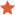## Prior Learning Assessment Course Subjects

### mathematical

More's indicate a better match.

Courses 1-3 of 3 matches.
History of Mathematics   (MAT-301)   3.00 s.h.Course Description
This course surveys the historical development of mathematics. Mathematical pedagogy, concepts, critical thinking and problem solving are studied from a historical perspective. The course aims at serving the needs of a wide student audience as well as connecting the history of mathematics to other fields such as the sciences, engineering, economics and social sciences. The course explores the major themes in mathematics history: arithmetic, algebra, geometry, trigonometry, calculus, probability, statistics and advanced mathematics. The historical development of these themes is studied in the context of various civilizations ranging from Babylonia and Egypt through Greece, the Far and Middle East, and on to modern Europe. Topics covered include ancient mathematics, medieval mathematics, early modern mathematics and modern mathematics.

Learning Outcomes
Through the Portfolio Assessment process, students will demonstrate that they can appropriately address the following outcomes:

• Apply mathematical techniques of problem solving.
• Describe the development of mathematics across and within civilizations around the world.
• Explain how different cultures have affected and been affected by the history of mathematics.
• Analyze and critically think about past, present and future mathematical problems.
• Recognize the distinction between formal and intuitive mathematics.
• Research historical mathematical concepts and present the conclusions of them.
• Compare and contrast the mathematical influences on the sciences, engineering, humanities and other fields.
• Present the history of mathematics in written forms.

Digital Signal Processing   (ELC-454)   3.00 s.h.Course Description
This course covers principles and knowledge required to successfully develop cost-effective digital signal processing solutions to problems related to such areas as controls, telecommunications, speech/audio, instrumentation, image processing, and biomedicine.

Learning Outcomes
Through the Portfolio Assessment process, students will demonstrate that they can appropriately address the following outcomes:

• Discuss principles of mathematical theorems used in digital signal processing
• Describe applications of digital signal processing to communications
• Describe applications of digital signal processing to control problems
• Provide evidence of programming for digital signal processors
• Identify hardware vs. software solutions for digital signal processing

Math and Science For Child   (CDS-271)   3.00 s.h.Course Description
This course identifies and classifies the major mathematical and science concepts and topics considered in teaching the young child. Emphasis is placed on planning Math and Science activities that encourage thinking, exploring, discovering and problem solving. Each concept is exemplified by hands-on experience.

Learning Outcomes
Through the Portfolio Assessment process, students will demonstrate that they can appropriately address the following outcomes:

• Discuss the cognitive and developmental capabilities of early childhood students in the areas of math and science.
• Address various philosophical approaches to the teaching of math and science.
• Indicate instructional activities that support both critical thinking and problem-solving.
• Give examples of hands-on experiences in both the math and science content areas.
• Discuss the challenges in planning developmentally appropriate math and science lessons/activities.
• You may address math and science together or as separate content-areas in the narrative paper.

Courses 1-3 of 3